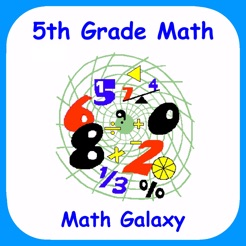Screenshots

•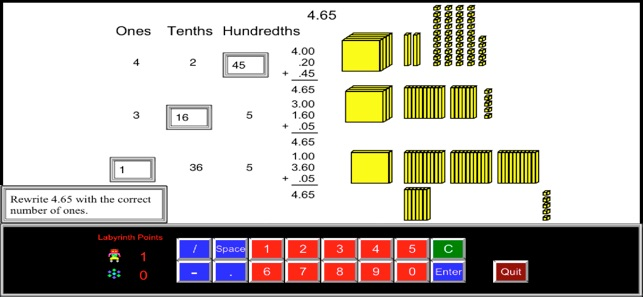•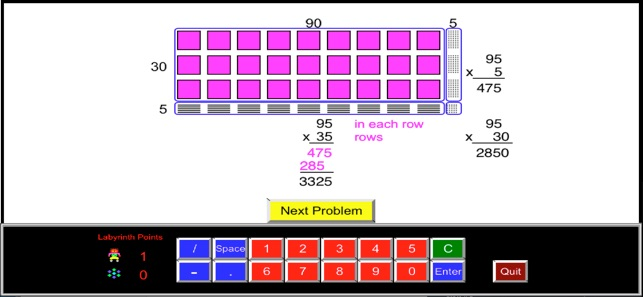•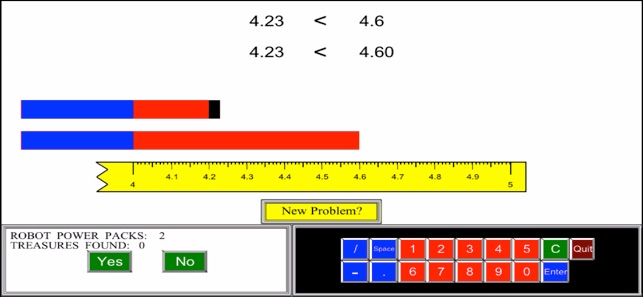•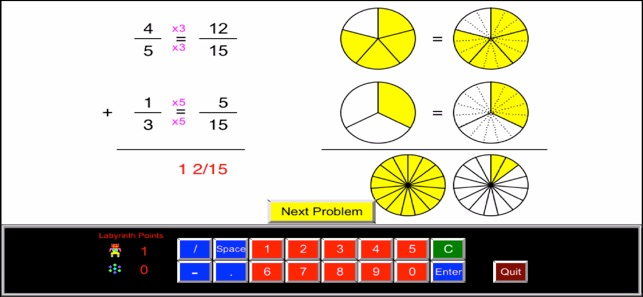•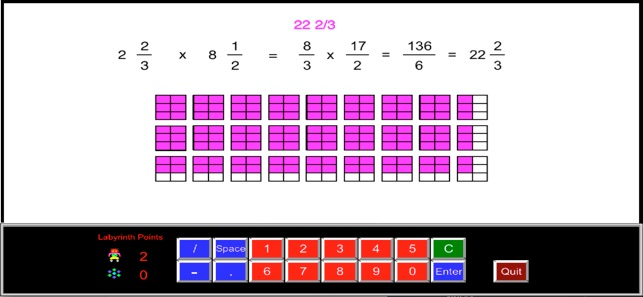•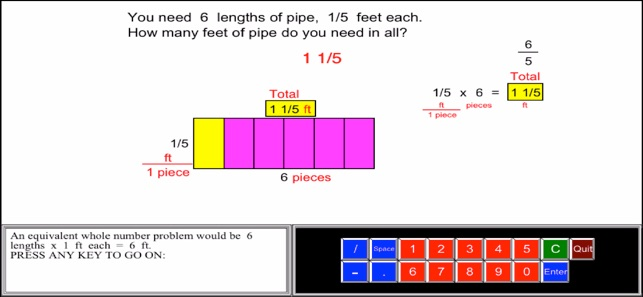•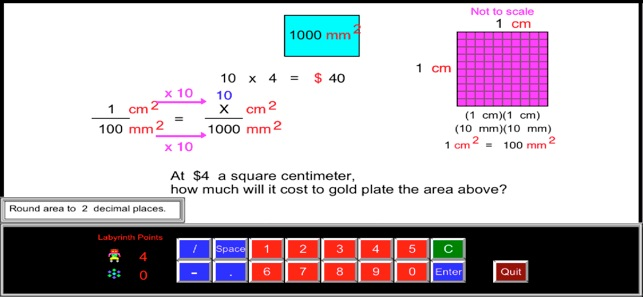•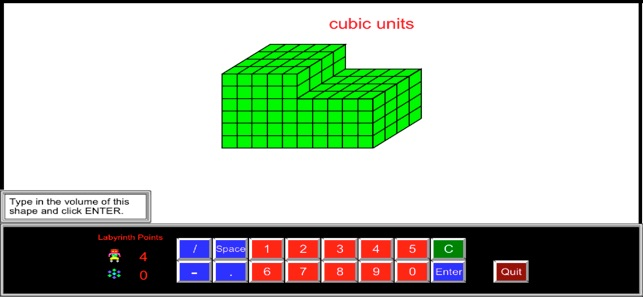•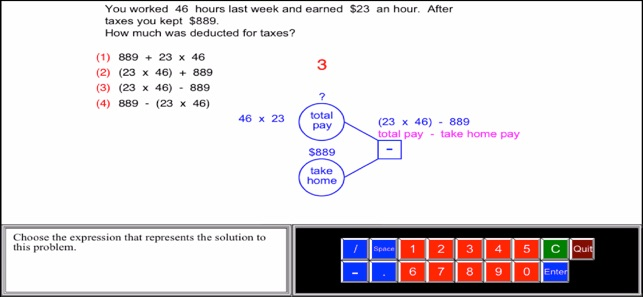•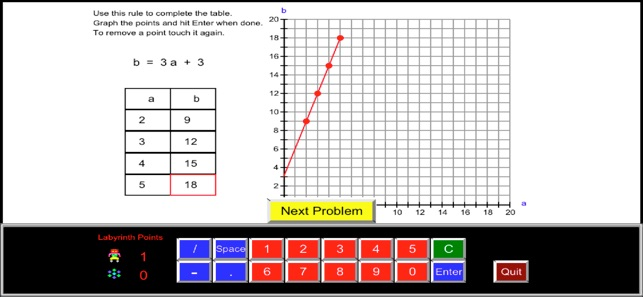Description

"5th Grade Math by Math Galaxy is a comprehensive review and practice app covering all of the basics for the grade level. This easy to navigate app offers kids a great way to get that extra little bit of help that they might need to solidify their understanding of basic math concepts."

"We have been able to find each and every math concept that we have been presented with this year outlined neatly within this app."

See the rest of the review at:

In fifth grade math the emphasis is on fractions, decimals and volume: (1) developing fluency with addition and subtraction of fractions and multiplication and division of fractions and whole numbers, (2) decimal operations to hundredths and whole number division with 2-digit divisors, and (3) volume.

Math Galaxy’s Fifth Grade Math covers all of the Common Core math standards for fifth grade as listed below.
The concepts are presented in bite-size pieces with an unlimited supply of practice problems, with numbers chosen randomly.
Illustrations are used throughout to reinforce the concepts.
By solving problems students earn robots to use in a maze game, plus there are several other games involving math practice.
Certificates and summaries of scores can be emailed to be printed.

NUMBERS AND OPERATIONS IN BASE 10 (5.NBT)

Understand the place value system.
Perform operations with multi-digit whole numbers, and with decimals to hundredths.

Place Value (5.NBT.1, 5.NBT.3a)
Place Names
Expanded Notation

Regrouping (5.NBT.1)
(Whole #s & Decimals)

Comparing Decimals (5.NBT.3b)

Rounding Decimals (5.NBT.4)

Multiplying & Dividing by 10s (5.NBT.2)
Multiply and Divide Taking Out Factors of 10 (5.NBT.1,2)

Multiplying Whole Numbers (5.NBT.5)
(2-3 Digit, Traditional & Alternative)

Dividing Whole Numbers (5.NBT.6)
(2-3 Digit Divisors, Traditional & Alternative)

Decimal Operations (5.NBT.7)
Subtracting
Multiplying
Dividing
Word Problems

FRACTIONS (5.NF)

Use equivalent fractions as a strategy to add and subtract fractions.
Apply and extend previous understandings of multiplication and division to multiply and divide fractions.

Adding & Subtracting (5.NF.1)
Multiplying Fractions (5.NF.4)
Fractions as Scaling (5.NF.5)
Dividing Unit Fractions (5.NF.7)
Dividing Simple Fractions (5.NF.7)
Dividing Mixed Numbers (5.NF.7)
Fraction Word Problems (5.NF.2, 6, 7c)
Fractions as Division (5.NF.3)
Multiply or Divide
All Operations

MEASUREMENT AND DATA (5.MD)

Convert like measurement units within a given measurement system.
Understand concepts of volume and relate volume to multiplication and addition.

Length (5.MD.1)
Area (5.MD.1)
Mass (5.MD.1)
Volume
Converting Units (5.MD.1)
Cubic Units 1 (5.MD.3, 5.MD.4)
Cubic Units 2 (5.MD.5c)
Missing Side (5.MD.5a, 5b)
Line Plots (5.MD.2)

GEOMETRY (5.G)

Graph points on the coordinate plane.
Classify two-dimensional figures into categories based on their properties.

Graphing Coordinates (5.G1, 5.G2)
Lines, Angles, & Polygons (5.G.3, 5.G.4)

ALGEBRAIC THINKING (5.OA)

Write and interpret numerical expressions.
Analyze patterns and relationships.

Algebraic Operations (5.OA.1, 5.OA.2)
Translating to Algebra (5.OA.2)
Order of Operations (5.OA..1)
Algebraic Patterns (5.OA.3)

GAMES

Riddles (Length, Area, Volume, & Time - in Measurement section)
Labyrinth (Guide robots through mazes in search of treasures.
Robots are earned by solving problems.)
The Money Trail
Bridge the Swamp (adding, subtracting, multiplying, & dividing fractions and decimals)

What’s New

Version 3.1

Fixed videos freezing.

Ratings and Reviews

3.0 out of 5
6 Ratings

6 Ratings

erika,. ,

I Don’t recommend this app... it never works

Nemesis Kate ,

Nice game for practicing math

Good game for improving your calculation and math skills, even gives some geometry theory. Some tasks are in a form of game where you can play with other player or with the computer. Every task allows you to earn points - robots, and then you can play a labyrinth game :) the only thing, it's really hard to tap using fingers.. As i saw they recommend stylus, but i think not everyone has it and maybe they should adapt it somehow for fatter fingers :) but my overall impression is really good, as this app is much better for kids than playing some angry birds!

John Karter from Mars :-) ,

Highly recommended

My son has become obviously better in fractions and volume after spending a few evening playing this app. It's fantastic! Thanks for this app, it's absolutely worth its money

Information

Seller
Gilbert Reilly
Size
196.6 MB
Category
Education
Compatibility

Requires iOS 8.0 or later. Compatible with iPhone, iPad, and iPod touch.

Languages

English

Age Rating
Rated 4+
© Math Galaxy 2017 (Gilbert Reilly)
Price
\$4.99

Supports

•